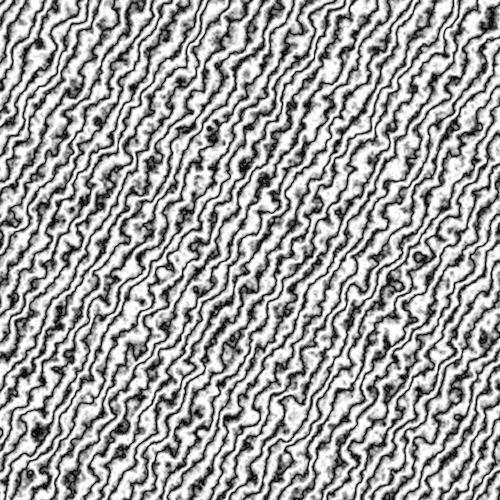# Landscape waves

P5.js, Law of Multistable-perception, Law of Emotion, form, grid
Random lines with different grey scale colors built a landscape.Lines built a landscape with different grey color depths. Looks like a Satellite picture of a Landscape or ocean.

``````var power = 3;
var d = 16;

function setup() {
var canvas = createCanvas(500, 500);
canvas.parent('sketch');
background(23,254,21);
}

function draw() {
for(var y = 0; y < height; y++) {
for (var x = 0; x < width; x++) {
var total = 0;
for (var i = d; i>= 1; i = i/2){
total += noise(x/d, y/d) * d;
}
var turbulence = 128 * total / d;
var base = (x * 0.2) + (y * 0.12);
var offset = base + (power * turbulence / 256);
var gray = abs(sin(offset)) * 256;
stroke(gray);
point(x, y);
}
}
}

``````